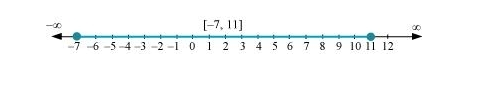# Solve the inequalities and represent the solution graphically on number line: 5(2x – 7) – 3(2x + 3) ≤ 0, 2x + 19 ≤ 6x + 47

Question:

Solve the inequalities and represent the solution graphically on number line: 5(2x – 7) – 3(2x + 3)  0, 2x + 19  6x + 47

Solution:

$5(2 x-7)-3(2 x+3) \leq 0$

$\Rightarrow 10 x-35-6 x-9 \leq 0$

$\Rightarrow 4 x-44 \leq 0$

$\Rightarrow 4 x \leq 44$

$\Rightarrow x \leq 11 \ldots(1)$

$2 x+19 \leq 6 x+47$

$\Rightarrow 19-47 \leq 6 x-2 x$

$\Rightarrow-28 \leq 4 x$

$\Rightarrow-7 \leq x \ldots(2)$

From (1) and (2), it can be concluded that the solution set for the given system of inequalities is [–7, 11]. The solution of the given system of inequalities can be represented on number line as